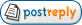Topic: Power in HRVAuthor Message
angel61606

Joined: Jul 8, 2020
Posts: 85Posted: Feb 20, 2022 10:28 AM    Post subject: Power in HRV Hi Jarek, to calculate power for vlf , lf and hf subbands of hrv i do as following:I have a design in which I am using RRDetector element, and then i use a Time resampler on its way and I send the output signal with rate 2Hz.then i use an stream to vector elemnt with size of 1024 and then i use FFT2Transform to calculate its fft ,then I calculate the magnitude of it. next, the subvectors regarding to each vlf , lf,hf is extracted and then sum of squares in each subvector is calculated.but somehow my output powers are way too smaller than the actual numbers. i have no idea why? do u have any suggestion? am i missing something? should i consider physical number? i am very clueless. thanks in advance.